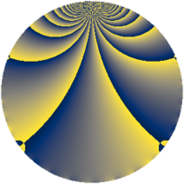# Properties

 Label 3630.2.beLevel $3630$ Weight $2$ Character orbit 3630.be Rep. character $\chi_{3630}(199,\cdot)$ Character field $\Q(\zeta_{22})$ Dimension $1320$ Sturm bound $1584$

# Related objects

## Defining parameters

 Level: $$N$$ $$=$$ $$3630 = 2 \cdot 3 \cdot 5 \cdot 11^{2}$$ Weight: $$k$$ $$=$$ $$2$$ Character orbit: $$[\chi]$$ $$=$$ 3630.be (of order $$22$$ and degree $$10$$) Character conductor: $$\operatorname{cond}(\chi)$$ $$=$$ $$605$$ Character field: $$\Q(\zeta_{22})$$ Sturm bound: $$1584$$

## Dimensions

The following table gives the dimensions of various subspaces of $$M_{2}(3630, [\chi])$$.

Total New Old
Modular forms 8000 1320 6680
Cusp forms 7840 1320 6520
Eisenstein series 160 0 160

## Trace form

 $$1320q + 132q^{4} + 8q^{5} - 4q^{6} - 1320q^{9} + O(q^{10})$$ $$1320q + 132q^{4} + 8q^{5} - 4q^{6} - 1320q^{9} - 18q^{10} + 84q^{11} - 8q^{14} - 4q^{15} - 132q^{16} + 16q^{19} - 8q^{20} - 8q^{21} + 4q^{24} + 4q^{25} + 8q^{26} + 8q^{30} - 8q^{34} + 24q^{35} - 132q^{36} - 8q^{39} - 4q^{40} - 40q^{41} + 4q^{44} - 8q^{45} - 16q^{46} + 220q^{49} + 16q^{50} + 8q^{51} + 4q^{54} - 36q^{55} + 8q^{56} + 40q^{59} + 4q^{60} - 56q^{61} + 132q^{64} + 188q^{65} + 4q^{66} - 12q^{70} + 80q^{71} - 8q^{74} + 16q^{75} - 60q^{76} + 72q^{79} + 8q^{80} + 1320q^{81} + 8q^{84} - 136q^{85} - 32q^{86} - 24q^{89} + 18q^{90} - 16q^{91} - 176q^{94} - 4q^{96} - 84q^{99} + O(q^{100})$$

## Decomposition of $$S_{2}^{\mathrm{new}}(3630, [\chi])$$ into newform subspaces

The newforms in this space have not yet been added to the LMFDB.

## Decomposition of $$S_{2}^{\mathrm{old}}(3630, [\chi])$$ into lower level spaces

$$S_{2}^{\mathrm{old}}(3630, [\chi]) \cong$$ $$S_{2}^{\mathrm{new}}(605, [\chi])$$$$^{\oplus 4}$$$$\oplus$$$$S_{2}^{\mathrm{new}}(1210, [\chi])$$$$^{\oplus 2}$$$$\oplus$$$$S_{2}^{\mathrm{new}}(1815, [\chi])$$$$^{\oplus 2}$$